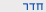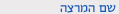שנה"ל תש"ףמבוא להסתברות וסטטיסטיקה
Intr. to Probability and Statistics
0509-2801-10
הנדסה | תואר ראשון - קורסי שירותסמ'  קיץ 0900-1200 'ב 102 הנדסה כתות ח שיעור ד"ר משיח אילה סמ'  קיץ 0900-1200 'ג 102 הנדסה כתות ח שיעור סמ'  קיץ 0900-1200 'ה 102 הנדסה כתות ח שיעור
ש"ס:  4.0

סילבוס מקוצר
משקל:                     3.5
דרישות קדם:         חשבון דיפרנציאלי ואינטגרלי.
קומבינטוריקה. מרחב מדגם ומאורעות, פונקצית הסתברות. הסתברות מותנית ומשפט בייס. משתנים אקראיים, פילוג ופילוג מותנה במאורע. תוחלת, שונות, אי שוויונות מרקוב וצ'בישב, מומנטים, פונקציה אופיינית. פלוגים: בינומי, גאומטרי, פואסוני, מעריכי, גאוסי. פונקציה של משתנה אקראי. שני משתנים אקראיים: התפלגות משותפת ושולית, פונקציות של שני משתנים אקראיים. מומנטים משותפים, פונקציה אופיינית משותפת, פילוג מותנה, תוחלת מותנית, משפט התוחלת. וקטור אקראי. סדרה אקראית. החוק החלש של המספרים הגדולים. משפט הגבול המרכזי. שערוך פרמטרים: רווח סמך לתוחלת, הגישה הבייסיאנית, סבירות מקסימלית, חסם קרמן ראו. בדיקת השערות.
Course description
Credit points: 3.5
Prerequisites: Differential and Integral Calculus.
Combinatorics, Sample space and events, probability function, conditional probability and Bayes theorem, Random Variables, distribution and conditional distribution, expectation and variance. Markov and Chebyshev inequalities, moments, characteristic function, distributions: Binomial. Geometric, Poison, Exponential. Gaussian, Functions of random variables, Bivariate distributions: joint and marginal distributions, functions of two random variables, joint moments, joint characteristic function, conditional distribution and conditional expectation, expectation theorem. Random vector, random series, weak law of large numbers, central limit theorem, parameters estimation, confidence intervals for the expected value, Bayesian approach, maximum likelihood, Rau Cramer inequality, tests of hypothesis.הנדסה | תואר ראשון - קורסי שירות
0509-2801-10 מבוא להסתברות וסטטיסטיקה
Intr. to Probability and Statistics
שנה"ל תש"ף | סמ'  קיץ | ד"ר משיח אילה

סילבוס מפורט/דף מידע
Biostatistics for biomedical engineers

Dr. Galit Ashkenazi-Golan

Objectives of the course: At the end of this course students should be able to (i) apply statistical methods to practical biomedical engineering problems; (ii) understand statistical designs of experiments; (iii) analyze and interpret data using statistical methods.
Main textbook:
"Engineering Statistics" (4th Edition, 2007), by D.C. Montgomery, G.C. Runger and N.F. Hubele, John Wiley & Sons, NY.
"Primer of Biostatistics" (5th Edition, 2002), by S. Glantz, McGraw Hill.
Course contents:
Contents
1 Basics of probability: Probability Space, Dependent and independent events, Poisson process, Bayes' theorem

2+3+4 Basics of probability (cont.): Random variables, Expectation, Variance, Common Distributions, Covariance, Pearson Correlation, Normal (Gaussian) distribution, exponential distribution, t-distribution, chi-square distribution, F-distribution

5+6 Concepts of descriptive statistics: Properties of random samples with size n, , point and interval estimators, confidence intervals identifying and rejecting outliers, boxplots

7 Testing of hypotheses:
basic concepts: type I and type II errors, significance and the p-value, 1-tail and 2-tail tests, decision making

8 Midterm
9+10 Testing of hypotheses (cont.): Z-test, T-test for one population, paired versus unpaired tests, testing the variance; examples relevant to biomedical research.

11 Systematic comparisons of means and variances: Testing for equality of variances, one-way ANOVA

12 Linear regression: The minimal sum of squared errors criterion, goodness of fit tests, Pearson correlation, examples relevant to biomedical research
13 Two-way ANOVA: testing with interactions, alpha corrections for multiple comparisons, power tests
14 Introduction to use of statistical software packages and practical implementation (SPSS, MATLAB statistics toolbox)
sizes